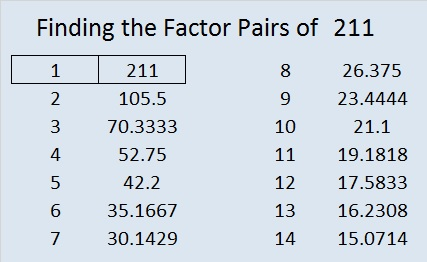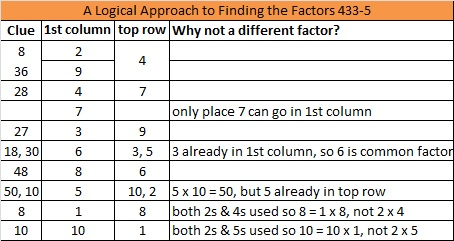# 211 and Level 5

• 211 is a prime number.
• Prime factorization: 211 is prime.
• The exponent of prime number 211 is 1. Adding 1 to that exponent we get (1 + 1) = 2. Therefore 211 has exactly 2 factors.
• Factors of 211: 1, 211
• Factor pairs: 211 = 1 x 211
• 211 has no square factors so its square root cannot be simplified. √211 ≈ 14.526How do we know that 211 is a prime number? If 211 were not a prime number, then it would be divisible by at least one prime number less than or equal to √211 ≈ 14.526. Since 211 cannot be divided evenly by 2, 3, 5, 7, 11, or 13, we know that 211 is a prime number.

Excel file of puzzles and previous week’s factor solutions: 10 Factors 2014-08-18This site uses Akismet to reduce spam. Learn how your comment data is processed.